Deutsche VersionRelative leveldecibel  chart
and
dB conversion calculator

Conversion of voltage or power  ratios to decibels dB
Table and chart − volts, watts, and pascals, W/m2

The dB calculator - a valuable tool

The sound pressure variation is the instantaneous acoustic pressure as RMS value.

 Voltage orsoundpressureratio Powerorintensityratio ← − dB + → Voltage orsoundpressureratio Powerorintensityratio 1.000 1.000 0 1.000 1.000 0.989 0.977 0.1 1.012 1.023 0.977 0.955 0.2 1.023 1.047 0.966 0.933 0.3 1.035 1.072 0.955 0.912 0.4 1.047 1.096 0.944 0.891 0.5 1.059 1.122 0.933 0.871 0.6 1.072 1.148 0.923 0.851 0.7 1.084 1.175 0.912 0.832 0.8 1.096 1.202 0.902 0.813 0.9 1.109 1.230 0.891 0.794 1.0 1.122 1.259 0.841 0.708 1.5 1.189 1.413 0.794 0.631 2.0 1.259 1.585 0.750 0.562 2.5 1.334 1.778 0.707 0.501 3.0 1.413 1.995 0.668 0.447 3.5 1.496 2.239 0.631 0.398 4.0 1.585 2.512 0.596 0.355 4.5 1.679 2.818 0.562 0.316 5.0 1.778 3.162 0.531 0.282 5.5 1.884 3.548 0.501 0.250 6.0 1.995 4.000 0.473 0.224 6.5 2.113 4.467 0.447 0.200 7.0 2.239 5.012 0.422 0.178 7.5 2.371 5.623 0.398 0.159 8.0 2.512 6.310 0.376 0.141 8.5 2.661 7.079 0.355 0.126 9.0 2.818 7.943 0.335 0.112 9.5 2.985 8.913 0.316 0.100 10 3.162 10.00 0.282 0.0794 11 3.55 12.6 0.251 0.0631 12 3.98 15.8 0.224 0.0501 13 4.47 20.0 0.199 0.0398 14 5.01 25.1 0.178 0.0316 15 5.62 31.6 0.159 0.0251 16 6.31 39.8 0.141 0.0200 17 7.08 50.1 0.126 0.0159 18 7.94 63.1 0.112 0.0126 19 8.91 79.4 0.100 0.0100 20 10.0 100.0 3.16×10−2 10−3 30 3.16×10 103 10−2 = 0.01 10−4 40 102 = 100 104 3.16×10−3 10−5 50 3.16×102 105 10−3 = 0.001 10−6 60 103 = 1000 106 3.16×10−4 10−7 70 3.16×103 107 10−4 10−8 80 104 108 3.16×10−5 10−9 90 3.16×104 109 10−5 10−10 100 105 1010 3.16×10−6 10−11 110 3.16×105 1011 10−6 10−12 120 106 1012
 To use the calculator, simply enter a value. The calculator works in both directions of the ↔ sign.

 Voltage ratio   V ratio = V2/V1 ↔ Voltage level LV  dB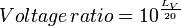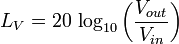Voltage ratio 1 ≡ 0 dB at reference Vin = 1 volt
 Power ratio   P ratio = P2/P1 ↔ Power level LP  dB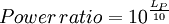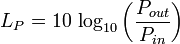Power ratio 1 ≡ 0 dB at reference Pin = 1 watt

For dBm it was decided the reference power P1 or P0 = 1 milliwatt = 0.001 watt (≡ 0 dB).

 Electric power (telephone) P   watts ↔ Electric Power level LP  dBm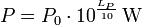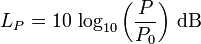Reference power P0 = 1 milliwatt (mW) = 0.001 W ≡ 0 dBm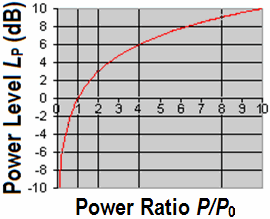Level of field quantities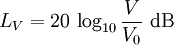Level of energy quantities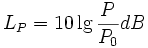We are mainly concerned with conditions of sound pressure, sound velocity, and audio voltage that are field quantities. Voltage level is: LV = 20 × log10(V1 / V0) Voltage is: V1 = V0 × 10(LV / 20) Reference voltage V0 = 1 Volt.

 Sound Field Quantities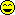Sound pressure, sound or particle velocity, particle displacement or displacement amplitude, (voltage, current, electric resistance). Inverse Distance Law 1/r Sound Energy Quantities Sound intensity, sound energy density, sound energy, acoustic  power. (electrical power). Inverse Square Law 1/r²

 Decibel scale for linear field quantities, like volts and sound pressures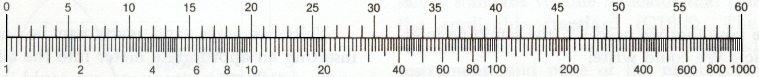The logarithmic scale (ratio)

Comparison of sound pressure level SPL and sound intensity level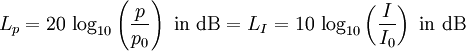Absolute level − dB chart

referred to 0.7746 volt in dBu and to 1 volt in dBV

Conversion of decibel to voltage re 0.7746 volt and re 1 volt

 Voltage re 0.7746 volt Voltage re 1 volt ← − dB + → Voltage re 0.7746 volt Voltage re 1 volt 0.775 1.000 0 0.775 1.000 0.766 0.989 0.1 0.784 1.012 0.757 0.977 0.2 0.793 1.023 0.748 0.966 0.3 0.802 1.035 0.740 0.955 0.4 0.811 1.047 0.731 0.944 0.5 0.820 1.059 0.723 0.933 0.6 0.830 1.072 0.715 0.923 0.7 0.840 1.084 0.706 0.912 0.8 0.849 1.096 0.698 0.902 0.9 0.859 1.109 0.690 0.891 1.0 0.869 1.122 0.652 0.841 1.5 0.921 1.189 0.615 0.794 2.0 0.975 1.259 0.581 0.750 2.5 1.033 1.334 0.548 0.707 3.0 1.095 1.414 0.518 0.668 3.5 1.159 1.496 0.489 0.631 4.0 1.228 1.585 0.461 0.596 4.5 1.300 1.679 0.436 0.562 5.0 1.377 1.778 0.411 0.531 5.5 1.459 1.884 0.388 0.501 6.0 1.549 1.995 0.367 0.473 6.5 1.637 2.113 0.346 0.447 7.0 1.734 2.239 0.327 0.422 7.5 1.837 2.371 0.308 0.398 8.0 1.946 2.512 0.291 0.376 8.5 2.061 2.661 0.275 0.355 9.0 2.183 2.818 0.259 0.335 9.5 2.312 2.985 0.245 0.316 10 2.449 3.162 0.218 0.282 11 2.748 3.548 0.195 0.250 12 3.084 3.981 0.173 0.224 13 3.460 4.467 0.155 0.199 14 3.882 5.012 0.138 0.178 15 4.356 5.623 0.123 0.159 16 4.887 6.310 0.109 0.141 17 5.484 7.079 0.097 0.129 18 6.153 7.943 0.086 0.112 19 6.904 8.912 0.077 0.100 20 7.746 10.000 2.450×10−2 3.162×10−2 30 24.50 31.62 7.746×10−3 10−2 40 77.46 102 2.450×10−3 3.162×10−3 50 2.450×102 3.162×102 7.746×10−4 10−3 60 7.746×102 103 2.450×10−4 3.162×10−4 70 2.450×103 3.162×103 7.746×10−5 10−4 80 7.746×103 104 2.450×10−5 3.162×10−5 90 2.450×104 3.162×104 7.746×10−6 10−5 100 7.746×104 105 2.450×10−6 3.162×10−6 110 2.450×105 3.162×105 7.746×10−7 10−6 120 7.746×105 106

Audio voltage and level

 To use the calculator, simply enter a value. The calculator works in both directions of the ↔ sign.

 Voltage V (audio)   volts ↔ Voltage level Lu  dBu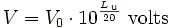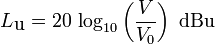Reference voltage V0 = 0.7746 Volt ≡ 0 dBu
 Voltage V   volts ↔ Voltage level LV  dBV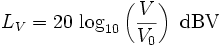Reference voltage V0 = 1 Volt ≡ 0 dBV
 Note - Comparing dBSPL and dBA:             There is no conversion formula for measured dBA             values to sound pressure level dBSPL or vice versa.             That is only possible measuring one single frequency.   There is no "dBA" curve given as threshold of human hearing.  Readings of a pure 1 kHz tone should be identical, whether weighted or not.

 Pro audio equipment often lists an A-weighted noise spec  – not because it correlates well with our hearing – but because it  can "hide" nasty hum components that make for bad noise specs.    Words to bright minds: Always wonder what a manufacturer   is hiding when they use A-weighting. *)

Sound measuring (Noise measuring) with weighting filter A and C

 If the output voltage level is 0 dB, that is 100%, the level of −3 dB is equivalent to 70.7% and the level of −6 dB is equivalent to 50% of the initial output voltage. This applies to all field quantities; e.g. sound pressure.   If the output power level is 0 dB, that is 100%, the level of −3 dB is equivalent to 50% and −6 dB is equivalent to 25% of the initial output power. This applies to all energy quantities; e.g. sound intensity.   Try to understand this.

Different equations in books −
RMS or peak value?

 The sound intensity I in W/m2 in a plane progressive wave is given as:or also as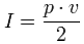But only one equation can be correct.   Sometimes, these equations will show further information: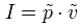or also as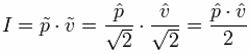The tilde will indicate that it is the RMS value and the roof will show that it is the amplitude value, ie, the peak value. For sinusoidal signals, the peak value means the amplitude. With these more accurate data, both equations are correct. You just need to know exactly whether the peak value or the RMS value is applied.   Sound pressure p in Pa = N/m2 − particle velocity v in m/s − acoustic intensity I in N/m2 · m/s = W/m²               Energy equivalent: J (joule) = N · m = W · s   In audio engineering we always assume RMS values, if not specially noted different.

 Simple rule of thumb: When working with power, 3 dB is twice, 10 dB is 10 times. When working with voltage or current, 6 dB is twice, 20 dB is 10 times